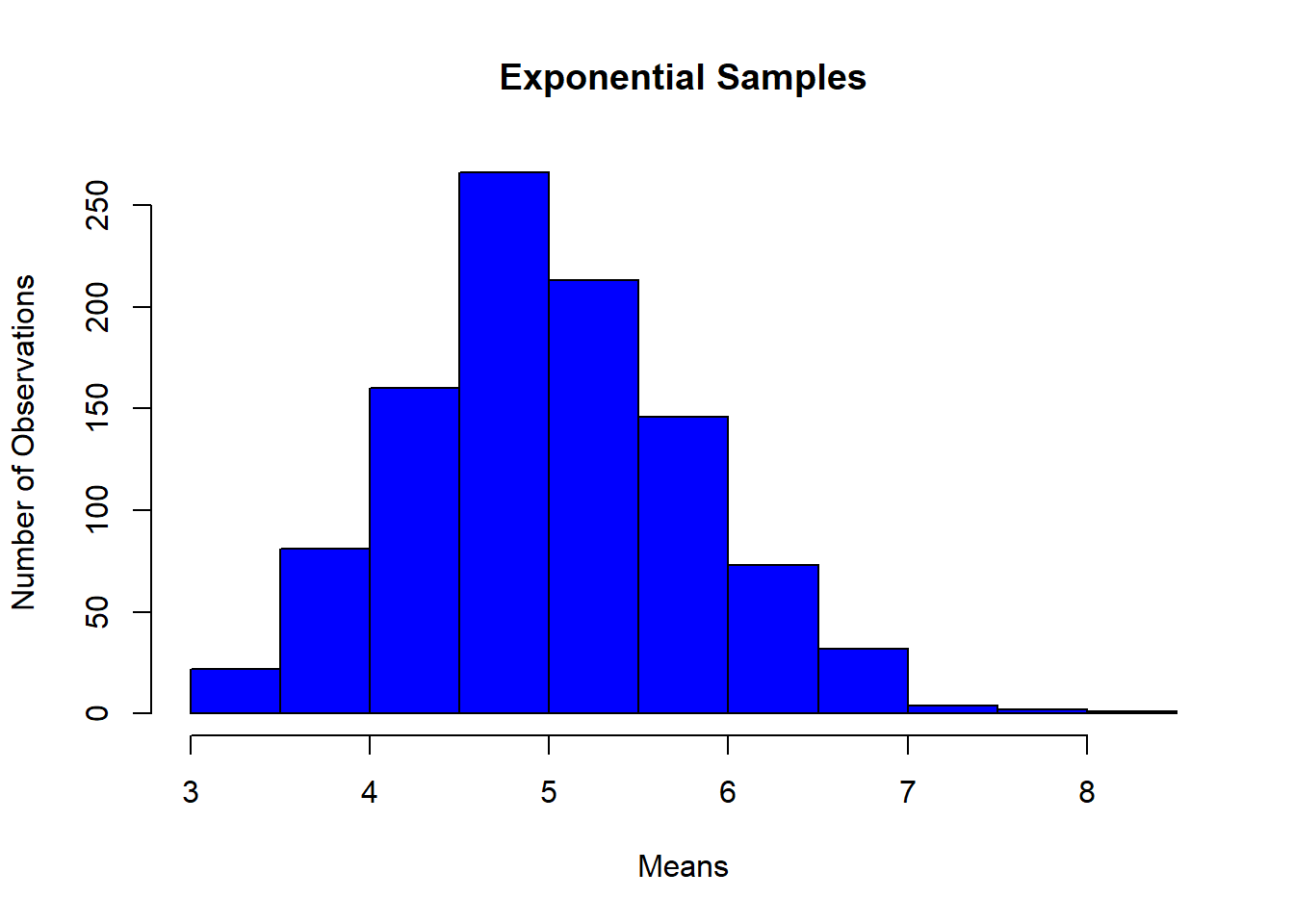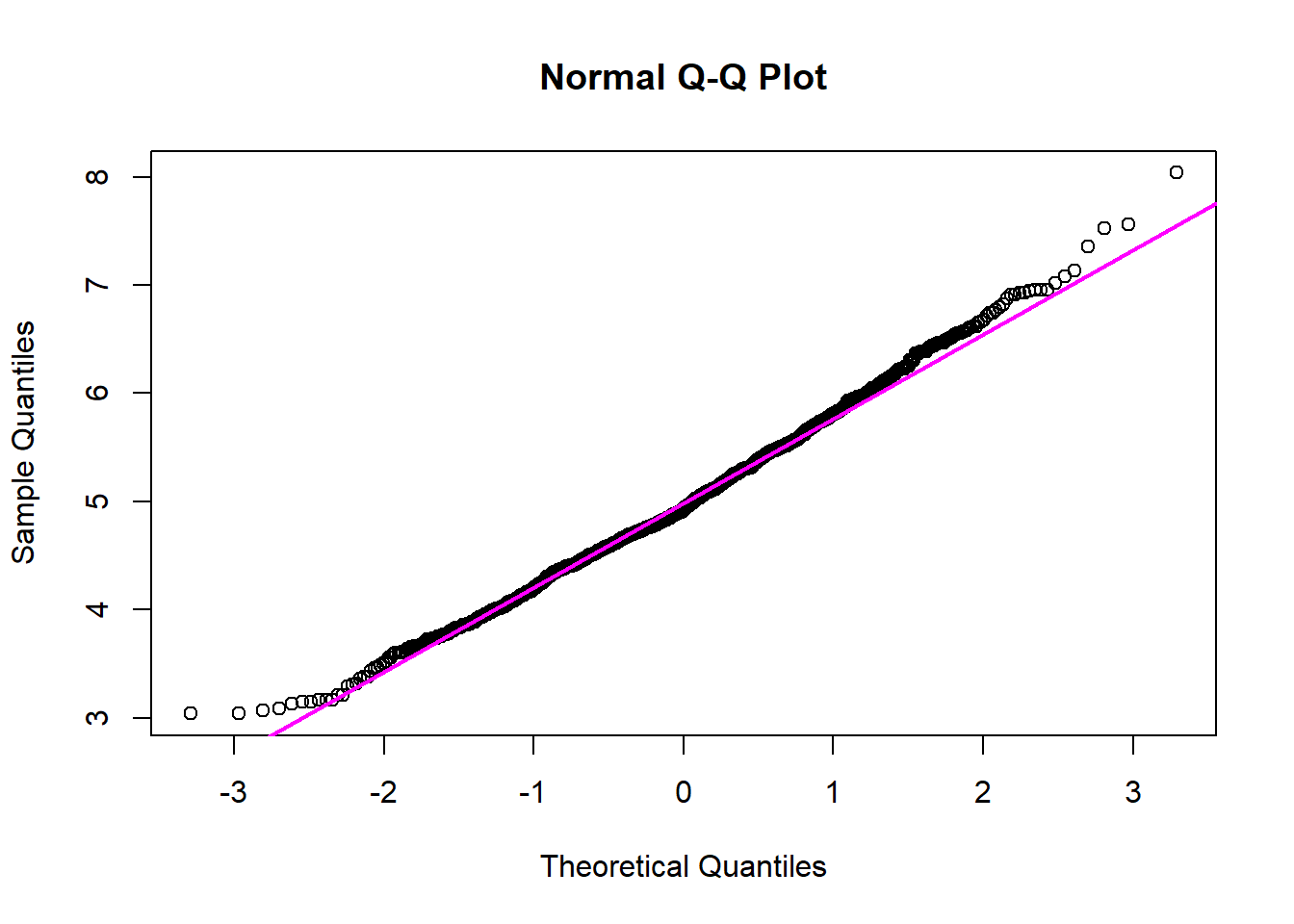## Simulation Exercise

### Overview

In this project, I will investigate the exponential distribution in R and compare it with the Central Limit Theorem (CLT). I will use the R function rexp(n, lambda) with n = 40 and lambda = 0.2. The mean of exponential distribution is 1/lambda and the standard deviation is also 1/lambda.

### Simulations

The CLT states that the distribution of averages of iid variables (properly normalized) becomes that of a standard normal as the sample size increases. I will run 1000 simulations of an exponential distribution to inductively prove this theorem to be true.

First, I will set the parameters for the exponential distribution as the assignment requests.

set.seed(100)
n <- 40
lambda <- 0.2

Next, I will do a panel plot showing the individual expMeans of one thousand simulations of the exponential distribution versus one thousand simulations of a uniform distribution.

expMeans = NULL
for (i in 1:1000) expMeans = c(expMeans, mean(rexp(n, lambda)))

Finally, I will construct a panel plot showing one thousand simulations of the exponential distribution versus one thousand simulations of a random uniform distribution.

hist(expMeans, main = "Exponential Samples",
xlab = "Means",
ylab = "Number of Observations",
col = "blue",
breaks = 10)### Sample Mean versus Theoretical Mean

In this section, I will show where the distribution is actually centered compared to the theoretical center of the distribution. The center of the distribution is more commonly referred to as the mean of the distribution.

First, I will calculate the mean of the sample using the mean() function in R.

# Sample Mean
sMean <- mean(expMeans)

The theoretical mean of an exponential distribution is defined as 1 / lambda. Remember, that we are using a value of 0.2 for lambda.

# Theoretical Mean
tMean <- 1 / lambda

Now, I will calculate the difference between the sample mean and the theoretical mean to show how close they are quantitatively.

diffMean <- abs(tMean - sMean)

The difference between the sample mean and the theoretical mean is, which is a relatively small value.

### Sample Variance versus Theoretical Variance

In this section, I will show how variable the distribution is and compare that variance to teh theoretical variance of the distribution.

First, I will calculate the standard deviation of the sample using the sd() function in R.

# Sample Standard Deviation
sSD <- sd(expMeans)

The theoretical standard deviation of an exponential distribution is defined as 1 / lambda. Remember, that we are using a value of 0.2 for lambda.

# Theoretical Standard Deviation
tSD <- 1 / lambda

Now, I will calculate the difference between the sample stanard deviation and the theoretical standard deviation to show how close they are quantitatively. Since the standard deviation is defined as the variance quantity squared, then we can get an accurate account of how varied the data are from one another this way.

diffSD <- abs(tSD - sSD)

The difference between the sample standard deviation and the theoretical standard devition is , which is a relatively small number.

### Distribution

In this section, I will give a visual explanation of why this distribution is approximately normal. I will use the R function qqnorm tp produce a normal QQ plot of the means of the exponential distribution that we got from the previous simulation. I will plot that against the theoretical normal quantile-quantile plot using the R function qqline.

# Comparison line to a normal distribution
qqnorm(expMeans)
qqline(expMeans, col = "magenta", lwd = 2)Notice how close the two lines are, and also that the variation between the variations on the tails of the lines is very small. This variation could have been even smaller if more simulations were run. This shows that the distribution of the means from the exponential distribution is approximately normal.